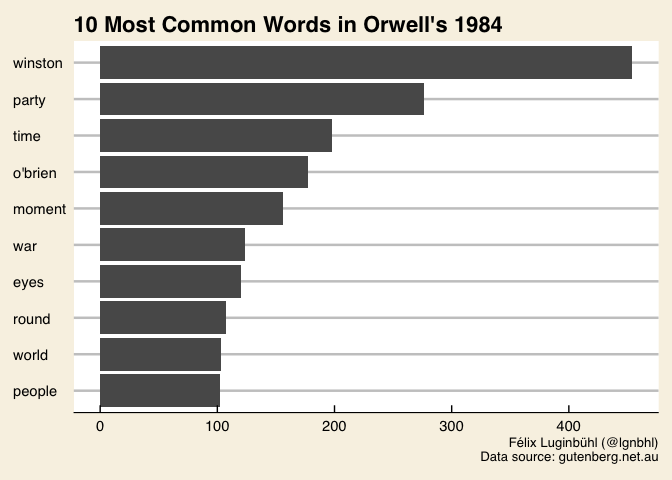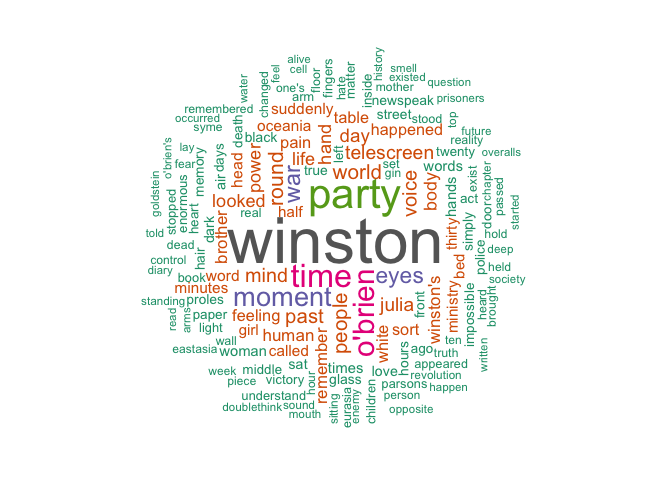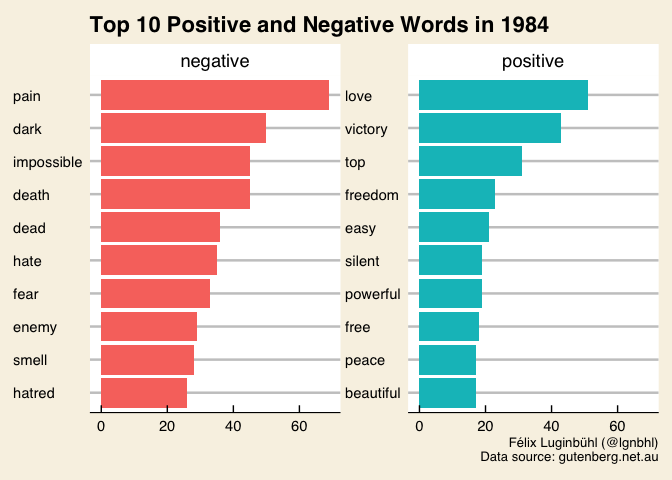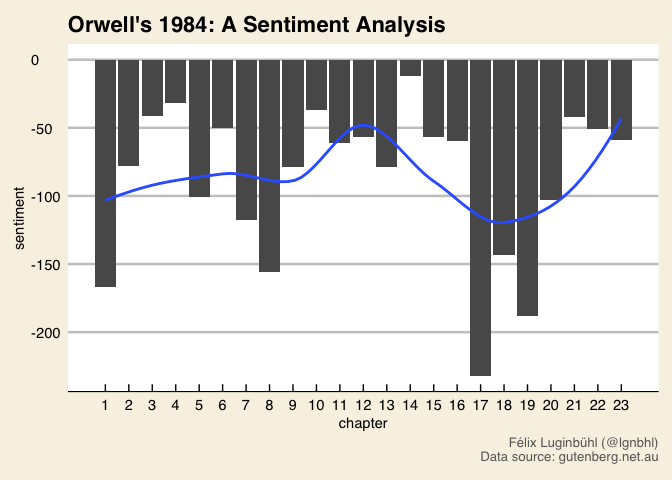# Orwell’s 1984, An (Un)Sentimental Analysis

Dystopian books are trendy. After Donald Trump’s election, George Orwell novel 1984 hit the No. 1 spot in Amazon’s book sales chart. Also because I love this book, let’s make a text analysis using the R package {tidytext}.

1. What are the words most frequently used?
2. What are the top negative and positive words?
3. Is a sentiment analysis informative?

## The Data

The novel 1984 is in the public domain in Canada and Australia (but neither Europe nor the US). We can find a text version of the book from the Projet Gutenberg Australia website here.

Following the ebook Text Mining with R from Julia Silge and David Robinson, let’s begin by tidying the text dataset.

library(tidyverse)
library(tidytext)

skip_empty_rows = TRUE,
skip = 38, # remove metadata
n_max = 8500) %>% # remove appendix
data_frame(text = .) %>%
write_csv(path = "input/text_1984.csv")

bigBro <- text_1984 %>%
unnest_tokens(word, text, format = "text") %>%
anti_join(stop_words)

## The Words of Orwell’s 1984

What are the 10 most frequent words used in 1984?

library(ggthemes)

bigBro %>%
count(word, sort = TRUE) %>%
filter(n > 100) %>%
mutate(word = reorder(word, n)) %>%
ggplot(aes(word, n)) +
geom_col() + coord_flip() +
theme_economist_white(gray_bg = F) +
theme(plot.background = element_rect(fill = "#f8f2e4")) +
labs(x = NULL, y = NULL,
title = "10 Most Common Words in Orwell's 1984",
caption = "Félix Luginbühl (@lgnbhl)\nData source: gutenberg.net.au")The two main characters “Winston” and “O’Brien” appear. We also get the main topics of Orwell’s totalitarian society with the words “party”, “time”, “war”, “eyes” and “people”.

The word “time” is the third most common word in 1984. Working at the Ministry of Truth, Winston rewrites the records of the past. His duty is to follow the party’s ever-changing version of history.

What about the other frequently used words in the book? A wordcloud can do the job nicely.

library(wordcloud)

bigBro %>%
count(word) %>%
with(wordcloud(word, n, max.words = 150, rot.per=0.35,
random.order = FALSE,
colors=brewer.pal(8, "Dark2")))In the wordcloud, we see the words “brother” (for Big Brother) and “Julia”, Winston’s love interest and other main characters of the novel. Other important words, like “telescreen”, “oceania”, “pain” or “human”, also appear.

## Top Negative and Positive Words

In order to identify positive and negative words, we need to use a sentiment dictionary. The package {tidytext} gives us access to three of them. Let’s apply the Bing sentiment lexicon from Bing Liu and collaborators to our text and visualize the top 10 negative/positive words in 1984.

bigBro %>%
inner_join(get_sentiments("bing")) %>%
count(word, sentiment, sort = TRUE) %>%
group_by(sentiment) %>%
top_n(10) %>%
ungroup() %>%
mutate(word = reorder(word, n)) %>%
ggplot(aes(word, n, fill = sentiment)) +
geom_col(show.legend = FALSE) +
facet_wrap(~sentiment, scales = "free_y") +
coord_flip() +
theme_economist_white() +
theme(plot.background = element_rect(fill = "#f8f2e4")) +
labs(x = NULL, y = NULL,
title = "Top 10 Positive and Negative Words in 1984",
caption = "Félix Luginbühl (@lgnbhl)\nData source: gutenberg.net.au")Although the word “love” appears 51 times, it is rarely linked with a positive sentiment. Indeed, the “Ministry of Love” is in charge of torturing political prisoners. We touch here the limits of the use of a sentiment lexicon.

## A Sentiment Analysis by Chapter

Sentiment words used in 1984 are mostly describing Winston’s emotions. So it makes sense to try a sentiment analysis. Let’s take the Text Mining with R’s sentiment analysis approach, defining a sentiment score for each chapter i of the book:

scorei = positivei - negativei

where positivei is the number of “positive” words in a chapter, and negativei the number of “negative” words in a chapter. For instance, if we have 100 positive words and 60 negative words in chapter 1, we get a score of 40.

First, we need to add a chapter variable in our dataset.

bigBro_2 <- read_csv("input/text_1984.csv") %>%
mutate(chapter = cumsum(str_detect(text, regex("^chapter [\\digit]",
ignore_case = TRUE)))) %>%
unnest_tokens(word, text, format = "text")

Now, let’s prepare our dataset for the sentiment analysis.

library(tidyr)

bigBro_sent <- bigBro_2 %>%
inner_join(get_sentiments("bing")) %>%
anti_join(stop_words) %>%
count(chapter, sentiment) %>%
spread(key = sentiment, value = n) %>%
mutate(sentiment = positive - negative)

print(bigBro_sent)
## Source: local data frame [23 x 4]
## Groups: chapter 
##
## # A tibble: 23 x 4
##    chapter negative positive sentiment
##      <int>    <int>    <int>     <int>
##  1       1      261       94      -167
##  2       2      118       40       -78
##  3       3       93       52       -41
##  4       4       80       48       -32
##  5       5      189       88      -101
##  6       6       80       30       -50
##  7       7      173       55      -118
##  8       8      264      108      -156
##  9       9      125       46       -79
## 10      10      105       68       -37
## # ... with 13 more rows

We are ready to run our final visualisation.

library(scales)

ggplot(bigBro_sent, aes(chapter, sentiment)) +
geom_col() +
scale_x_continuous(breaks = c(1:23)) +
geom_smooth(method = "loess", se = FALSE) +
theme_economist_white() +
theme(plot.background = element_rect(fill = "#f8f2e4"),
plot.caption = element_text(color = "dimgrey")) +
labs(title = "Orwell's 1984: A Sentiment Analysis",
caption = "Félix Luginbühl (@lgnbhl)\nData source: gutenberg.net.au")We see that the sentiment score of each chapter is negative. Our sentiment analysis reflects plainly the dark and pessimistic tone of the novel.

Let’s look at chapters 1, 8 and 17, which are the first chapters of the three parts dividing 1984. We notice that these first chapters get the worst score.

Our sentiment analysis also shows that the sentiment score of each chapter is generally improving until chapter 17. Those who read the novel know what happens to Winston in this final part of the book.